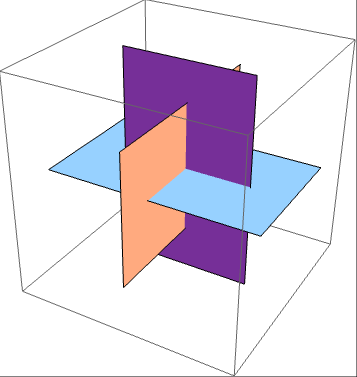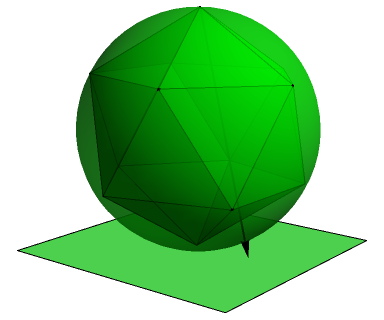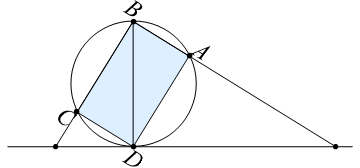As I mentioned a few posts ago, I included the Diophantine equation x^4 + y^6 = z^10 on the Advanced Mentoring Scheme. I’m not going to spoil it here, although I have since been informed that I had previously included it as an exercise in MODA.

Let’s consider the weaker equation, a^2 + b^3 = c^5. It’s quite easy to solve it using the good old Sam Cappleman-Lynes technique (henceforth abbreviated to SCLT), beginning with a solution to d^2 + e^3 = f. The simplest solution to this is 1 + 1 = 2, which we’ll then multiply throughout by 2^24 to give an integer solution to the original equation.

• 4096^2 + 256^3 = 32^5

Note that 4096, 256 and 32 are not coprime by any stretch of the imagination. Can we find a coprime solution to a^2 + b^3 = c^5 in the positive integers? I stumbled across an interesting discussion which demonstrated a geometrical construction for the Diophantine equation a^4 + b^3 = c^2. I’ll explain and apply it to the more flamboyant case of our equation, which is intimately connected to the geometry of the icosahedron.

### The geometry of the icosahedron

The twelve vertices of the icosahedron can be partitioned into three concentric congruent golden rectangles, the perimeters of which form Borromean rings:The side lengths of the rectangles are in the ratio 1 : φ, where φ = (1 + sqrt(5))/2 is the golden ratio. We’ll use this later. Firstly, note that the icosahedron can be inscribed in a sphere. We then identify this (the Riemann sphere) with the extended complex plane (like C, but with a point at infinity) by stereographic projection. This is another recurring theme in MODA.We can see instantly that the lowest vertex is at 0, and the one diametrically opposite is at ∞. There’s still a complex degree of freedom left, which we’ll choose carefully to give the neatest expressions for the remaining vertices. Let’s choose a pair of antipodal vertices to lie on the real line, and take a cross-section of the sphere:Let the diameter BD be 1. Then, we can deduce that the points on the horizontal line are positioned at ψ and φ, the two roots of z^2 − z − 1 = 0. By symmetry, we get that the twelve vertices are the roots of the projective equation xy(y^5 − φ^5 x^5)(y^5 − ψ^5 x^5) = 0, which expands out to f(x, y) = x y^11 − 11 x^6 y^6 − x^11 y = 0; the coefficient ’11’ comes from being the fifth Lucas number. Hence, plotting the roots of z^11 − 11 z^6 − z = 0 in Wolfram Alpha will give you the vertices of a stereographically projected icosahedron.### Hessians and Jacobians

Following the instructions in the e-mail, we compute the Hessian h and Jacobian j of f and h. These are the determinants of matrices of partial derivatives. After scaling to make the coefficients smaller, we get:

• h = x^20 − 228 x^15 y^5 + 494 x^10 y^10 + 228 x^5 y^15 + y^20
• j = x^30 + 522 x^25 y^5 − 10005 x^20 y^10 − 10005 x^10 y^20 −
522 x^5 y^25 + y^30

Amazingly, we have the identity h^3 − 1728 f^5 = j^2, which holds for all complex values of (x,y). (That’s not the only place where 1728 appears as a scaling factor!) We can recover a solution to the original equation by applying a subsitution:

• a = j
• b = −h
• c = 1728^(1/5) f

Now, these aren’t integer polynomials in x and y, so we rescale by letting x = 16^(1/5) s and y = 9^(1/5) t. If we substitute s = t = 1 we get a solution in the integers, but a couple of the values are negative. If we try s = 2 and t = 1 instead, the results are positive. This leads to a valid positive integer solution where a,b,c are coprime:

• 127602747389962225^2 + 196120763999^3 = 7506024^5

As an exercise, you might want to do the same with the octahedron to get a solution to a^2 + b^3 = c^4 with coprime terms. Enjoy!

### Beal’s conjecture

This is almost (but not quite) a counter-example to Beal’s conjecture. This claims that for a solution to a^l + b^m + c^n with l,m,n > 2, the integers a,b,c share a common factor. It is obvious that this (if true) implies Fermat’s last theorem, since from a solution to a^n + b^n = c^n we could divide throughout by gcd(a,b,c)^n to obtain a coprime solution. Not that this is of much interest, anyway, since we already know that Fermat’s last theorem is true.

Unfortunately, the geometric method cannot be applied to generate a solution with all exponents greater than 2, and SCLT just gives massive common factors. This is one of the many Annoying Aspects of Mathematics. Another example is that the only pairs of positive integers (m,n) for which 1² + 2² + 3² + … + m² = n² holds are (1, 1) and (24, 70), and it’s impossible to dissect a 70 by 70 square into squares of side lengths 1, 2, …, 24. Indeed, it’s an open problem with a \$100 prize as to whether there exists a rectangle which can be dissected into squares of side lengths 1, 2, …, m. By comparison, Beal’s conjecture holds a \$100000 prize.

This entry was posted in Uncategorized. Bookmark the permalink.

### 2 Responses to The inadequacy of SCLT

1.tomtom2357 says:

I get that the icosahedron (and analogously the octahedron and tetrahedron) have symmetry groups corresponding to a pair of generators with orders 2 and 3 and their product having order 5 (or 4 or 3 analogously). But how on earth does this translate to a solution to a^2+b^3=c^5? I can follow the algebra, but what I don’t get is why it works. What do the Hessians and Jacobians have to do with it? Could someone please demystify this seemingly magical construction?